• school Campus Bookshelves
• perm_media Learning Objects
• how_to_reg Request Instructor Account
• hub Instructor Commons
• Periodic Table
• Physics Constants
• Scientific Calculator
• Reference & Cite
• Tools expand_more

This action is not available.## 8.3: Concentrations of Solutions (Problems)

• Last updated
• Save as PDF
• Page ID 119753

PROBLEM $$\PageIndex{1}$$

What mass of a concentrated solution of nitric acid (68.0% HNO 3 by mass) is needed to prepare 400.0 g of a 10.0% solution of HNO 3 by mass?

PROBLEM $$\PageIndex{2}$$

What mass of a 4.00% NaOH solution by mass contains 15.0 g of NaOH?

PROBLEM $$\PageIndex{3}$$

What mass of solid NaOH (97.0% NaOH by mass) is required to prepare 1.00 L of a 10.0% solution of NaOH by mass? The density of the 10.0% solution is 1.109 g/mL.

$$\mathrm{114 \;g}$$

PROBLEM $$\PageIndex{4}$$

The hardness of water (hardness count) is usually expressed in parts per million (by mass) of $$\ce{CaCO_3}$$, which is equivalent to milligrams of $$\ce{CaCO_3}$$ per liter of water. What is the molar concentration of Ca 2+ ions in a water sample with a hardness count of 175 mg CaCO 3 /L?

$$1.75 \times 10^{−3} M$$

PROBLEM $$\PageIndex{5}$$

A throat spray is 1.40% by mass phenol, $$\ce{C_6H_5OH}$$, in water. If the solution has a density of 0.9956 g/mL, calculate the molarity of the solution.

PROBLEM $$\PageIndex{6}$$

Copper(I) iodide (CuI) is often added to table salt as a dietary source of iodine. How many moles of CuI are contained in 1.00 lb (454 g) of table salt containing 0.0100% CuI by mass?

$$\mathrm{2.38 \times 10^{−4}\: mol}$$

PROBLEM $$\PageIndex{7}$$

What are the mole fractions of H 3 PO 4 and water in a solution of 14.5 g of H 3 PO 4 in 125 g of water?

$$X_\mathrm{H_3PO_4}=0.021$$

$$X_\mathrm{H_2O}=0.979$$

PROBLEM $$\PageIndex{8}$$

What are the mole fractions of HNO 3 and water in a concentrated solution of nitric acid (68.0% HNO 3 by mass)?

$$X_\mathrm{HNO_3}=0.378$$

$$X_\mathrm{H_2O}=0.622$$

PROBLEM $$\PageIndex{9}$$

Calculate the mole fraction of each solute and solvent:

• 583 g of H 2 SO 4 in 1.50 kg of water—the acid solution used in an automobile battery
• 0.86 g of NaCl in 1.00 × 10 2 g of water—a solution of sodium chloride for intravenous injection
• 46.85 g of codeine, C 18 H 21 NO 3 , in 125.5 g of ethanol, C 2 H 5 OH
• 25 g of I 2 in 125 g of ethanol, C 2 H 5 OH

$$X_\mathrm{H_2SO_4}=0.067$$

$$X_\mathrm{H_2O}=0.933$$

$$X_\mathrm{HCl}=0.0026$$

$$X_\mathrm{H_2O}=0.9974$$

$$X_\mathrm{codiene}=0.054$$

$$X_\mathrm{EtOH}=0.946$$

$$X_\mathrm{I_2}=0.035$$

$$X_\mathrm{EtOH}=0.965$$

PROBLEM $$\PageIndex{10}$$

• 0.710 kg of sodium carbonate (washing soda), Na 2 CO 3 , in 10.0 kg of water—a saturated solution at 0 °C
• 125 g of NH 4 NO 3 in 275 g of water—a mixture used to make an instant ice pack
• 25 g of Cl 2 in 125 g of dichloromethane, CH 2 Cl 2
• 0.372 g of histamine, C 5 H 9 N, in 125 g of chloroform, CHCl 3

$$X_\mathrm{Na_2CO_3}=0.0119$$

$$X_\mathrm{H_2O}=0.988$$

$$X_\mathrm{NH_4NO_3}=0.09927$$

$$X_\mathrm{H_2O}=0.907$$

$$X_\mathrm{Cl_2}=0.192$$

$$X_\mathrm{CH_2CI_2}=0.808$$

$$X_\mathrm{C_5H_9N}=0.00426$$

$$X_\mathrm{CHCl_3}=0.997$$

PROBLEM $$\PageIndex{11}$$

What is the difference between a 1 M solution and a 1 m solution?

In a 1 M solution, the mole is contained in exactly 1 L of solution. In a 1 m solution, the mole is contained in exactly 1 kg of solvent.

PROBLEM $$\PageIndex{12}$$

What is the molality of phosphoric acid, H 3 PO 4 , in a solution of 14.5 g of H 3 PO 4 in 125 g of water?

PROBLEM $$\PageIndex{13}$$

What is the molality of nitric acid in a concentrated solution of nitric acid (68.0% HNO 3 by mass)?

PROBLEM $$\PageIndex{14}$$

Calculate the molality of each of the following solutions:

• 0.710 kg of sodium carbonate (washing soda), Na 2 CO 3 , in 10.0 kg of water—a saturated solution at 0°C

6.70 × 10 −1 m

PROBLEM $$\PageIndex{15}$$

A 13.0% solution of K 2 CO 3 by mass has a density of 1.09 g/cm 3 . Calculate the molality of the solution.

## Contributors

• Adelaide Clark, Oregon Institute of Technology

Think one of the answers above is wrong? Let us know here .

## Chapter: 11th Chemistry : UNIT 9 : Solutions

Solved example problems: chemistry: solutions.

Chemistry: Solutions

Numerical Problems Questions with Answers, Solution

## Example Problem 1

1. What volume of 4M HCl and 2M HCl should be mixed to get 500 mL of 2.5 M HCl?

Let the volume of 4M HCl required to prepare 500 mL of 2.5 MHCl = x mL

Therefore, the required volume of 2M HCl = (500 - x) mL

We know from the equation (9.1)

C 1 V 1 + C 2 V 2  =   C 3 V 3

(4x)+2(500-x)       =       2.5 × 500

4x+1000-2x         =       1250

2x  = 1250 - 1000

x    = 250/2

= 125 mL

Hence, volume of 4M HCl required = 125 mL

Volume of 2M HCl required = (500 - 125) mL= 375 mL

## Example Problem 2 :

0.24 g of a gas dissolves in 1 L of water at 1.5 atm pressure. Calculate the amount of dissolved gas when the pressure is raised to 6.0 atm at constant temperature.

p solute  = K H x solute in solution

At pressure 1.5 atm,

p 1  = K H  x 1 ------------(1)

At pressure 6.0 atm,

p 2  = K H  x 2 ------------(2)

Dividing equation (1) by (2)

From equation     p 1 /p 2  = x 1 /x 2

1.5/6.0 = 0.24/x 2

Therefore x 2  = 0.24 x 6.0/1.5 = 0.96 g/L

## Example Problem 3:

An aqueous solution of 2 % nonvolatile solute exerts a pressure of 1.004 bar at the boiling point of the solvent. What is the molar mass of the solute when P A ° is 1.013 bar?In a 2 % solution weight of the solute is 2 g and solvent is 98 g

ΔP = P A °  – P solution = 1.013 -1.004 bar = 0.009 barM B  = 2 x 18 x 1.013/(98 x 0.009)

= 41.3 g mol -1

Example Problem 4

0.75 g of an unknown substance is dissolved in 200 g water. If the elevation of boiling point is 0.15 K and molal elevation constant is 7.5 K Kg mol -1  then, calculate the molar mass of unknown substance

ΔT b   = K b  m

= K b  x W 2  x 1000 / M 2  x W 1

M 2  = K b  x W 2  x 1000 / ΔT b  x W 1

= 7.5 x 0.75 x 1000 / 0.15 x 200

= 187.5 g mol -1

Example Problem 5

Ethylene glycol (C 2 H 6 O 2 ) can be at used as an antifreeze in the radiator of a car. Calculate the temperature when ice will begin to separate from a mixture with 20 mass percent of glycol in water used in the car radiator. K f  for water = 1.86 K Kg mol-1 and molar mass of ethylene glycol is 62 g mol -1.

Weight of solute (W 2 ) = 20 mass percent of solution means 20 g of ethylene glycol

Weight of solvent (water) W 1  = 100 -20 = 80 g

ΔT f  = K f mThe temperature at which the ice will begin to separate is the freezing of water after the addition of solute i.e 7.5 K lower than the normal freezing point of water (273-7.5K) = 265.5 K

## Example Problem-6 :

At 400K 1.5 g of an unknown substance is dissolved in solvent and the solution is made to 1.5 L. Its osmotic pressure is found to be 0.3 bar. Calculate the molar mass of the unknown substance.## Example Problem – 7

The depression in freezing point is 0.24K obtained by dissolving 1g NaCl in 200g water. Calculate van’t-Hoff factor. The molal depression constant is 1.86 K Kg mol -1

Molar mass of solute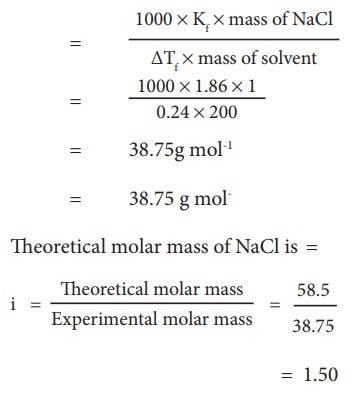40. A sample of 12 M Concentrated hydrochloric acid has a density 1.2 gL –1  Calculate the molality

Molality = 12 M HC l

density of the solution = 1.2 gL −1

In 12 M HC l  solution, there are 12 moles of HC l  in 1 litre of the solution

Molality = no. of moles of solute / mass of solvent (in Kg)

Calculate mass of water (solvent) mass of 1 litre HC l  solution = density × volume

= 1.2 gmL −1  × 1000 ml = 1200 g

mass of HC l  = no. of moles of HC l  × molar mass of HC l

= 12 mol × 36.5 g mol −1  = 438 g

mass of water = mass of HC l  solution − mass of HC l

mass of water = 1200 − 438 = 762 g

molality = 12 / 0.762

41. A 0.25 M glucose solution at 370.28 K has approximately the pressure as blood does what is the osmotic pressure of blood ?

T= 370.28 K

(π) glucose  = CRT

(π) = 0.25molL −1  × 0.082L atm K −1  mol −1  × 370.28K

42. Calculate the molality of a solution containing 7.5 g of glycine (NH 2 -CH 2  -COOH) dissolved in 500 g of water.

molality = no. of moles of solute / mass of solvent (in Kg)

no. of moles of glycine = mass of glycine / molar mass of glycine = 7.5 / 75 = 0.1

molality = 0.1 / 0.5 Kg = 0.2 m

43. Which solution has the lower freening point?10 g of methanol (CH 3 OH) in 100g of water (or) 20 g of ethanol (C 2 H 5 OH) in 200 g of water.

ΔT f  = K f  m ; ie ΔT f   ∝  m

m (CH3OH)  = (10/32) / 0.1 = 3.125m

M (C2H5OH)   = (20/46) / 0.2 = 2.174m

∴  depression in freezing point is more in methanol solution and it will have lower freezing point.

44. How many moles of solute particles are present in one litre of 10-4 M potassium sulphate?

In 10 -4  M K 2 SO 4  solution, there are 10 -4  moles of potassium sulphate.

K 2 SO 4  molecule contains 3 ions (2K +  and 1 SO 4 2−  )

1 mole of K 2 SO 4  contains 3 × 6.023×10 23  ions

10 -4  mole of K 2 SO 4  contains 3 × 6.023×10 23  × 10 −4  ions

= 18.069×10 19

45. Henry’s law constant for solubility of methane in benzene is 4.2x10 -5  mm Hg at a particular constant temperature At this temperature. Calculate the solubility of methane at i) 750 mm Hg ii) 840 mm Hg

(k H  ) benzene  = 4.2 × 10 -5  mm Hg ;

Solubility of methane = ?

P = 750mm Hg

P = 840 mm Hg

According to Henry's Law

P = K H . x  in solution

750mm Hg = 4.2 x 10 -5  mm Hg. x in solution

⇒   x in solution  = 750 / (4.2×10 -5 )

i.e. solubility, = 178.5 × 10 5  = 1.785 × 10 5

similarly at P = 840 mm Hg

solubility = 840 / 4.2×10 -5

= 200 × 10 -5

46. The observed depression in freezing point of water for a particular solution is 0.093ºC. Calculate the concentration of the solution in molality. Given that molal depression constant for water is 1.86 KKg mol -1

ΔT f  = 0.093°C = 0.093K ;

K f  = 1.86 K Kg mol −1  ;

ΔT f  = K f .m

∴  μ = ΔT f  / K f

m = 0.093K / 1.86 K Kg mol −1

= 0.05 mol Kg  −1  = 0.05 m.

47. The vapour pressure of pure benzene (C 6 H 6 ) at a given temperature is 640 mm Hg. 2.2 g of non-volatile solute is added to 40 g of benzene. The vapour pressure of the solution is 600 mm Hg. Calculate the molar mass of the solute?

P° C6H6  = 640 mm Hg

W 2  = 2.2 g (non volatile solute)

W 1  = 40 g (benzene)

P solution  = 600mm Hg

M 2  = ?

(P°−P) / P° = X 2  ;

(640 – 600) / 640 = n 2  / n 1  + n 2        [ ∵  n 1  >> n 2  ; n 1  + n 2  = n 1  ]

40 / 640 = n 2  / n 1

0.0625 = ( W 2  × M 1  ) / (M 2  × W 1 )

M 2  = (2.2 × 78) / (0.0625 × 40) = 68.64 g mol −1

Related Topics

## Chemistry Solutions Practice Problems## Molar solutions

To make a 1 M solution of sodium chloride, dissolve 58.44 g sodium chloride in 500 mL water in a 1000-mL volumetric flask. When all the solid is dissolved and the solution is at room temperature, dilute to the mark and invert the flask several times to mix.

To make a 2 M solution of acetic acid, dissolve 120.1 g acetic acid in 500 mL distilled or deionized water in a 1000-mL volumetric flask. Since acetic acid is a liquid, it may also be measured by volume. Divide the mass of acid by its density (1.049 g/mL) to determine the volume (114 mL). Use either 120.1 g or 114 mL acetic acid to make the solution. Swirl the flask gently to mix the solution. Once the solution is at room temperature, dilute to the mark and invert the flask several times to mix.

## Percent solutions

Since the solute (phenolphthalein) is a solid, the solution is percent by mass. Mass percent means the number of grams of solute per 100 g of solution.

mass percent = (mass of solute/mass of solution) x 100%

mass of solute = mass percent x mass of solution/100%

= 0.5% x 100 g/100%

Since the total mass of the solution equals 100 g, the remaining 99.5 g of the solution is water. To prepare the solution, dissolve 0.5 g phenolphthalein in 99.5 g distilled or deionized water.

Volume percent means the number of milliliters of solute per 100 mL of solution. Dilute 22 mL acetic acid with distilled or deionized water to make 100 mL of solution.

Calculate the volume of 3.0 M sulfuric acid needed to prepare the dilution.

M reagent  Ã—  V reagent  =  M dilution  Ã—  V dilution    3.0 M Ã—  V reagent  = 0.10 M Ã— 1.0 L   V reagent  = 0.033 L = 33 mL

Slowly add 33 mL of 3.0 M sulfuric acid to a 1000-mL volumetric flask half-filled with distilled or deionized water and swirl the flask to mix. Once the solution is at room temperature, dilute to the mark with water and invert the flask several times to mix.

Calculate the volume of 5.0 M sodium hydroxide needed to prepare the dilution.

M reagent  Ã—  V reagent  =  M dilution  Ã—  V dilution   5.0 M Ã—  V reagent  = 0.25 M Ã— 0.500 L    V reagent  = 0.025 L = 25 mL

Slowly add 25 mL of 5.0 M sodium hydroxide to a 500-mL volumetric flask half-filled with distilled or deionized water and swirl the flask to mix. Once the solution is at room temperature, dilute to the mark with water and invert the flask several times to mix.

## Special cases

Calculate the mass of impure potassium chloride needed.

mass of impure potassium chloride =  M pure  Ã—  V pure  Ã— gram formula weight / percent purity = 1.0 M Ã— 0.500 L Ã— 74.56 g/mol 0.930

Slowly add 40 g of 93% potassium chloride to a 500-mL volumetric flask half-filled with distilled or deionized water and swirl the flask to mix. When all the solid is dissolved and the solution is at room temperature, dilute to the mark and invert the flask several times to mix.

Calculate the volume of 85.0% phosphoric acid needed.

volume of impure phosphoric acid =  M pure  Ã—  V pure  Ã— gram formula weight / (percent purity x density) = (1.0 M Ã— 0.500 L x 98.00 g/mol) Ã· 1.685 g/mL 0.850

Slowly add 34 mL of 85.0% phosphoric acid to a 500-mL volumetric flask half-filled with distilled or deionized water and swirl the flask to mix. Once the solution is at room temperature, dilute to the mark with water and invert the flask several times to mix.

## Normal solutions

Magnesium hydroxide contains two hydroxyl groups. One-half mole of magnesium hydroxide, therefore, accepts one mole of protons. To prepare a 1.0 N solution of magnesium hydroxide, slowly add 29 g magnesium hydroxide to a 500-mL volumetric flask half-filled with distilled or deionized water and swirl the flask to mix. When all the solid is dissolved and the solution is at room temperature, dilute to the mark and invert the flask several times to mix.## carolinastaff

Carolina is teamed with teachers and continually provides valuable resources–articles, activities, and how-to videos–to help teachers in their classroom.

## Naming the Elements

Webinar: think like an engineer, you may also like, dimensional analysis, sea floor spreading-divergent plate boundaries, plate tectonics: evidence of plate movement, advantages of digital communication transmission, flame tests and spectroscopy: get excited about color, openscied, made even better by carolina, a model of interacting fields, wetland feedback loops, modeling river delta formation, which chemistry kit is right for you, leave a comment cancel reply.

Save my name, email, and website in this browser for the next time I comment.

• Biology Topics
• Dissection Resources
• Carolina Correlations
• Carolina 3D

## Get Started

• School Year Planning
• Carolina Essentials
• 800.334.5551
• [email protected]
• 2700 York Rd, Burlington, NC 27215-3398December 4, 2023

October 30, 2023

November 2, 2023

October 20, 2023

## Physical Science Math Review: Techniques, Formulae, and Constants

September 7, 2023

## One in a Million: Using Serial Dilutions to…

Basic right triangle trigonometry, how to use mathematics and logical routines.

August 25, 2023

October 5, 2023

July 27, 2023

## Why Don’t Whales Have Legs?

• NGSS & 3D Learning
• Workshops & Webinars
• Shop Carolina.com
• Lab Material Shopping Lists

This website uses cookies to improve your experience. We'll assume you're ok with this, but you can opt-out if you wish. Accept Read More

If you're seeing this message, it means we're having trouble loading external resources on our website.

If you're behind a web filter, please make sure that the domains *.kastatic.org and *.kasandbox.org are unblocked.

## AP®︎/College Chemistry

Course: ap®︎/college chemistry   >   unit 3.

• Types of mixtures

## Solutions and mixtures

• an integer, like 6 ‍
• a simplified proper fraction, like 3 / 5 ‍
• a simplified improper fraction, like 7 / 4 ‍
• a mixed number, like 1   3 / 4 ‍
• an exact decimal, like 0.75 ‍
• a multiple of pi, like 12   pi ‍   or 2 / 3   pi ‍

Subjects Home

## Chemistry 30

Practice Questions 2.2: Calculations Involving Solution Concentration    This worksheet is also available in the following formats:  Word | RTF | PDF

## Teacher Resources

Put this information together to solve the problem, arranging the information to end up with the desired unit:

Our final answer: [C 2 H 5 OH ] = 2.60M

What mass of NaCl are dissolved in 152 mL of a solution if the concentration of the solution is 0.364 M?

Because the question involves mass, we will need to know the molar mass of NaCl

Using a periodic table we find the molar mass of NaCl to be 58.5 g·mol -1

Because the question involves mass, we will need to know the molar mass of C 6 H 12 O 6

Using a periodic table we find the molar mass of C 6 H 12 O 6 to be 180.1 g·mol -1

Because the question involves mass, we will need to know the molar mass of H 2 SO 4

Using a periodic table we find the molar mass of H 2 SO 4 to be 98.1g·mol -1

L = 1 L 0.500 mol × 1 mol 98.1 g × 98.0 g = 2.00 L                 answer
Our final answer: [ Na 2 CO 3 ] = 2.33 M
Final answer: [HNO 3 ] = 3.93 × 10 -2 M

Because the question involves mass, we will need to know the molar mass of Cu(NO 3 ) 2

Using a periodic table we find the molar mass of Cu(NO 3 ) 2 to be 187.6 g·mol -1

Final answer: 4.27 × 10 -2 g of copper(II) nitrate are present.#### IMAGES

1. Solutions Problems: Chemistry 101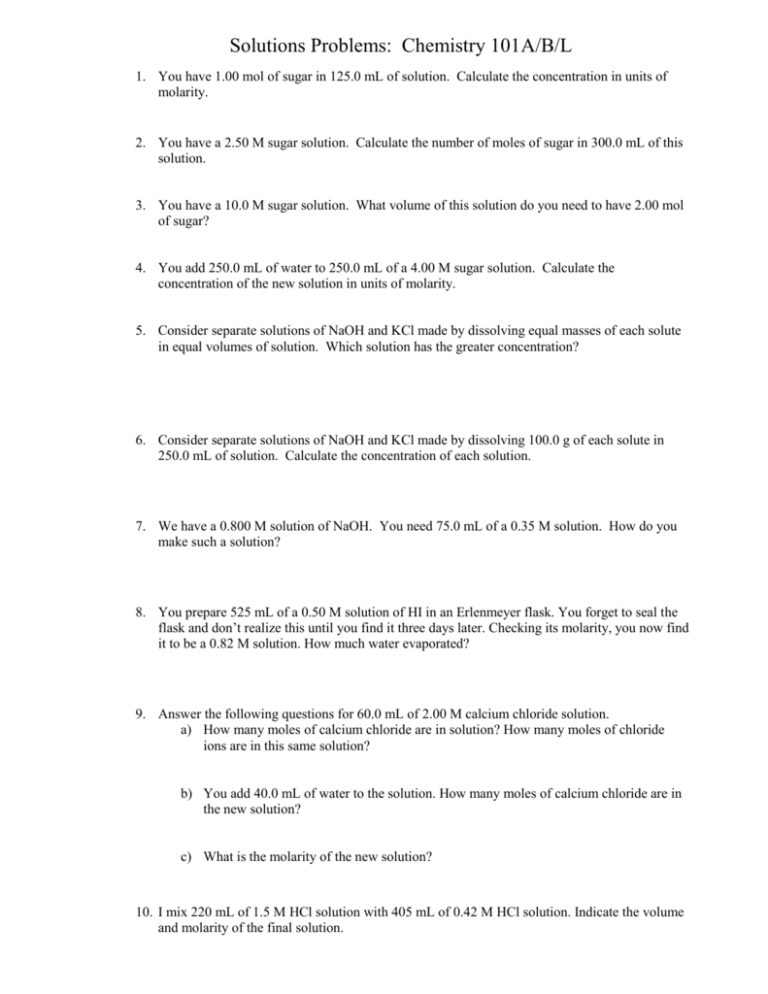2. Solved: Chapter 20E Problem 2P Solution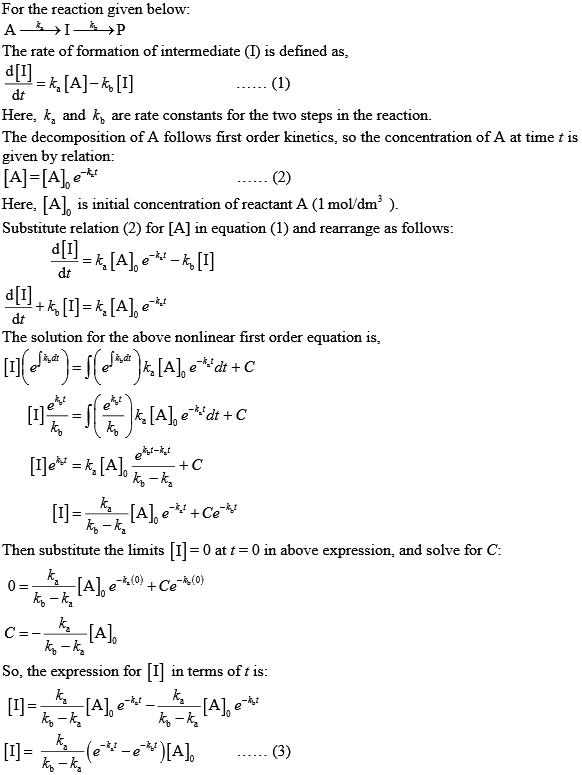3. Problems and Solutions in Organic Chemistry Oxford in pdf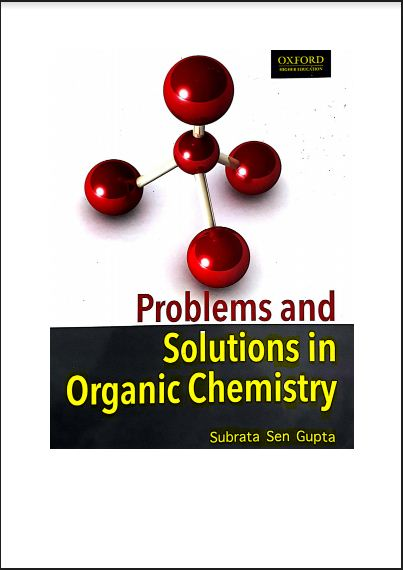4. Solved Example Problems: Chemistry: Solutions5. solutions problems chemistry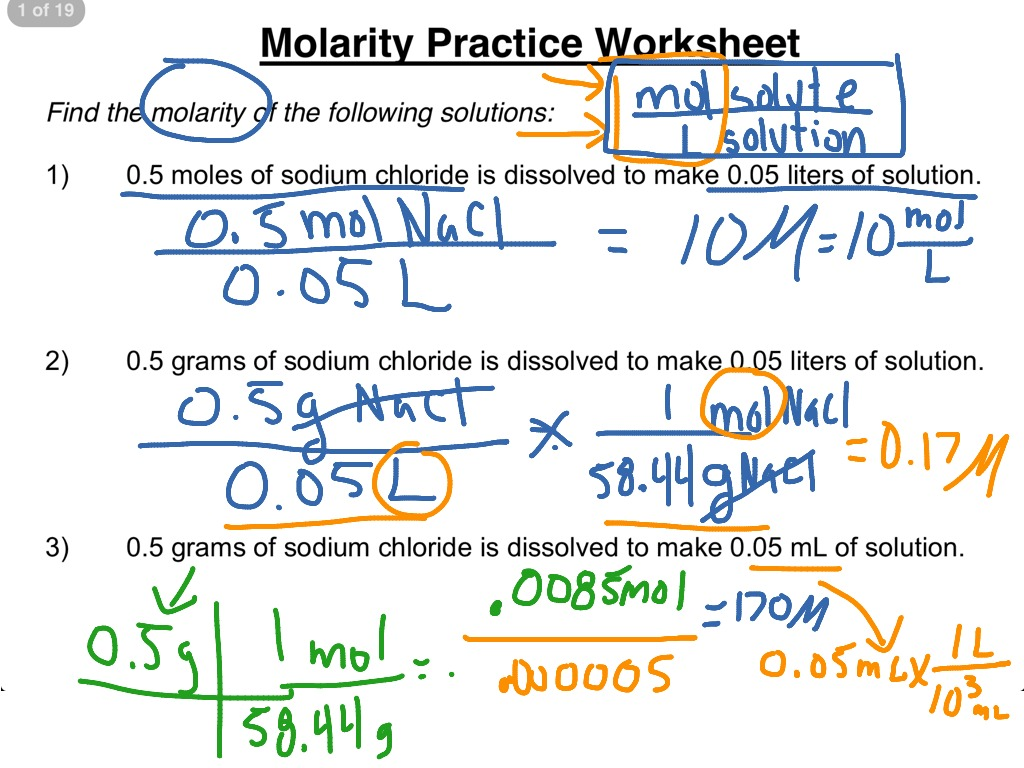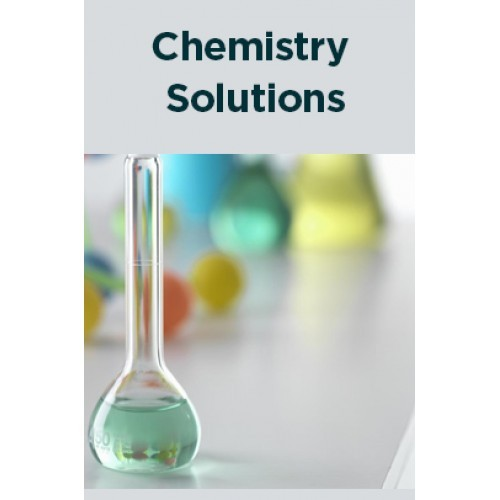#### VIDEO

1. problem of chemistry #shorts #chemistry

2. Learning by Solving Problems Chapter 11 1

3. 55 important chemical formula

4. exercise solving of some basic concept of chemistry Q 3 & 4 From A to F

5. chemistry class| chemistry problems| chemistry basics| learn chemistry fast| chemistry for beginners

6. Solutions and Mixtures

1. What Are Types of Solutions in Chemistry?

There are three types of solutions in chemistry: gaseous solutions, liquid solutions, and solid solutions. These are equivalent to the three main phases used in chemistry. Solutions are homogeneous mixtures, which means that the components ...

2. What Are the Branches of Environmental Science?

The branches of environmental science are ecology, atmospheric science, environmental chemistry, environmental engineering and geoscience. Environmental science is the study of the environment with a focus on providing solutions to environm...

3. How Is an Indicator Defined in Chemistry?

In chemistry, an indicator is defined as a substance that undergoes distinct observable change when the conditions of its solution change. Litmus is the most commonly used indicator in the laboratory.

4. 6.1.1: Practice Problems- Solution Concentration

What is the molarity of the solution? Answer. 11.9 M. PROBLEM 6.1.1.15. An experiment in a general chemistry laboratory calls for a 2.00-M

5. 8.3: Concentrations of Solutions (Problems)

8.3: Concentrations of Solutions (Problems) · 0.710 kg of sodium carbonate (washing soda), Na2CO3, in 10.0 kg of water—a saturated solution at 0

6. Dilution Problems, Chemistry, Molarity & Concentration ...

7. Solved Example Problems: Chemistry: Solutions

47. The vapour pressure of pure benzene (C6H6) at a given temperature is 640 mm Hg. 2.2 g of non-volatile solute is added to 40 g of benzene.

8. Chemistry Solutions Practice Problems

2. Describe how you would prepare 500 mL of a 1.0 M solution of phosphoric acid from 85.0% phosphoric acid that is pure. The gram formula weight of phosphoric

Atomic Structure Problems · Practice identifying atomic number, mass number, and atomic mass. · Get examples showing ways to find atomic mass. · Use Avogadro's

10. Solutions and mixtures (practice)

Do 4 problems. Check.

11. Page 1 of 7 SOLUTIONS CHEMISTRY PRACTICE PROBLEMS FOR

SOLUTIONS. CHEMISTRY PRACTICE PROBLEMS FOR NON-TECHNICAL MAJORS. Section 1: 1.1 Define mole, Avogadro's number, GAW, and GFW. In 500 grams of. 3. 4. Na PO , how

12. Chem 101 Solutions Lecture Problems

Solutions Practice Problems: Chemistry 101. 1. You have 1.00 mol of sugar in 125.0 mL of solution. Calculate the concentration in units of molarity. 2. You

13. Chemistry Problems and Solutions

01: UNITS OF MEASUREMENT ; 02: GASES ; 03: GAS MIXTURES AND OTHER PHYSICAL PROPERTIES OF ; : AVOGARDO'S HYPOTHESIS, CHEMICAL COMPOUNDS AND ; 05: STOICHIOMETRY /

14. Chemistry 30 Solution Chemistry Practice Question Answers

Solutions Practice Questions Answers. Practice Questions 2.2: Calculations Involving Solution Concentration This worksheet is also available in the following